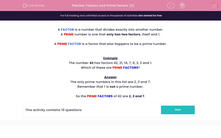# Factors and Prime Factors (2)

In this worksheet, students identify factors and prime factors of the given numbers.This content is premium and exclusive to EdPlace subscribers.Key stage:  KS 2

Curriculum topic:   Maths and Numerical Reasoning

Curriculum subtopic:   Special Numbers

Difficulty level:#### Worksheet Overview

A FACTOR is a number that divides exactly into another number.

A PRIME number is one that only has two factors, itself and 1.

A PRIME FACTOR is a factor that also happens to be a prime number.

Example

The number 42 has factors 42, 21, 14, 7, 6, 3, 2 and 1.

Which of these are PRIME FACTORS?

The only prime numbers in this list are 2, 3 and 7.

Remember that 1 is not a prime number.

So the PRIME FACTORS of 42 are 2, 3 and 7.

### What is EdPlace?

We're your National Curriculum aligned online education content provider helping each child succeed in English, maths and science from year 1 to GCSE. With an EdPlace account you’ll be able to track and measure progress, helping each child achieve their best. We build confidence and attainment by personalising each child’s learning at a level that suits them.

Get started